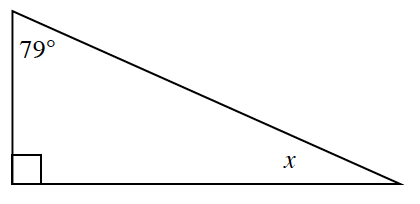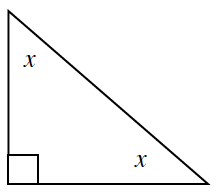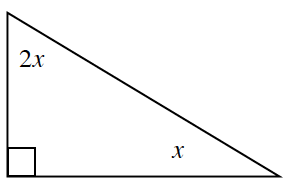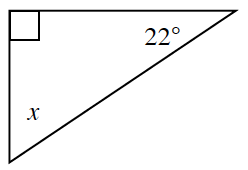### Home > INT2 > Chapter 3 > Lesson 3.2.1 > Problem3-72

3-72.

Use what you know about the angles of a triangle to determine the value of $x$ and the angle(s) in each triangle below.

Use the Triangle Angle Sum Theorem.

1.$x + 79^\circ + 90^\circ = 180^\circ$

1.$x + x + 90^\circ = 180^\circ$

1.$x = 30^\circ$

1.$x = 68^\circ$